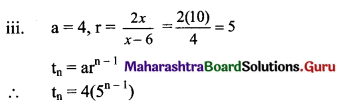# Maharashtra Board 11th Maths Solutions Chapter 2 Sequences and Series Ex 2.1

Balbharti Maharashtra State Board 11th Maths Book Solutions Pdf Chapter 2 Sequences and Series Ex 2.1 Questions and Answers.

## Maharashtra State Board 11th Maths Solutions Chapter 2 Sequences and Series Ex 2.1

Question 1.
Check whether the following sequences are G.P. If so, write tn.
(i) 2, 6, 18, 54, ……
Solution: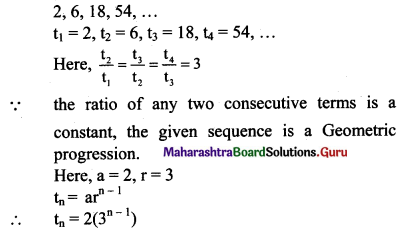(ii) 1, -5, 25, -125, ………
Solution: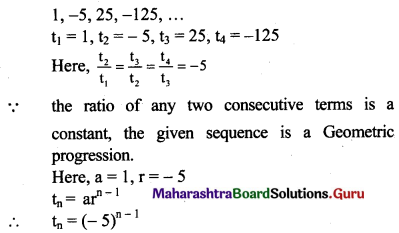(iii) $$\sqrt{5}, \frac{1}{\sqrt{5}}, \frac{1}{5 \sqrt{5}}, \frac{1}{25 \sqrt{5}}, \cdots$$
Solution: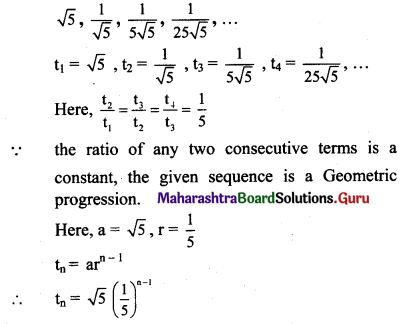(iv) 3, 4, 5, 6, ……
Solution: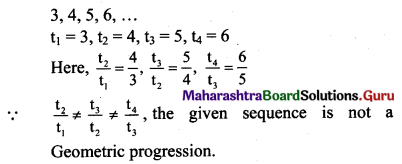(v) 7, 14, 21, 28, ……
Solution: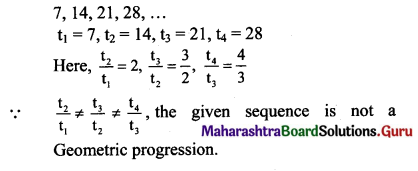Question 2.
For the G.P.
(i) If r = $$\frac{1}{3}$$, a = 9, find t7.
Solution: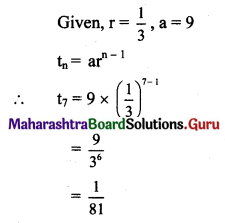(ii) If a = $$\frac{7}{243}$$, r = 3, find t6.
Solution: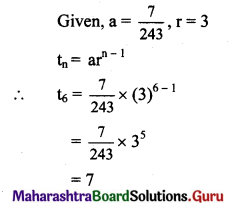(iii) If r = -3 and t6 = 1701, find a.
Solution: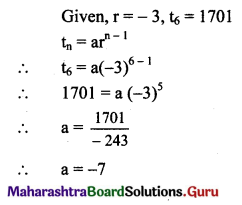(iv) If a = $$\frac{2}{3}$$, t6 = 162, find r.
Solution: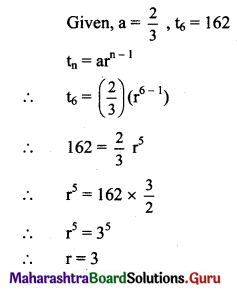Question 3.
Which term of the G. P. 5, 25, 125, 625, …… is 510?
Solution: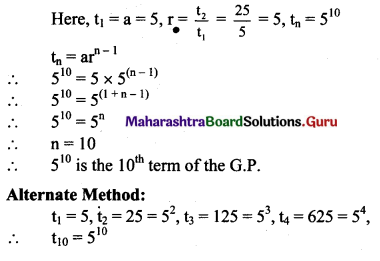Question 4.
For what values of x, the terms $$\frac{4}{3}$$, x, $$\frac{4}{27}$$ are in G. P.?
Solution: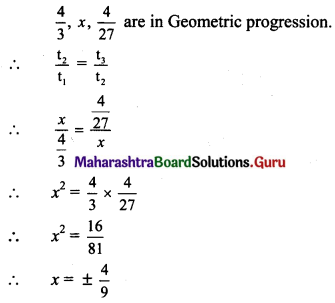Question 5.
If for a sequence, $$\mathrm{t}_{\mathrm{n}}=\frac{5^{n-3}}{2^{n-3}}$$, show that the sequence is a G. P. Find its first term and the common ratio.
Solution: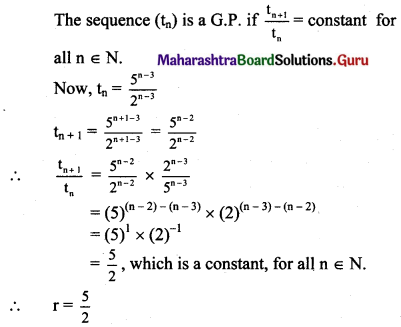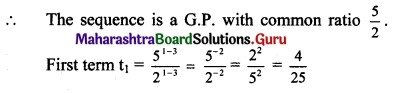Question 6.
Find three numbers in G. P. such that their sum is 21 and the sum of their squares is 189.
Solution:
Let the three numbers in G. P. be $$\frac{a}{r}$$, a, ar.
According to the given conditions,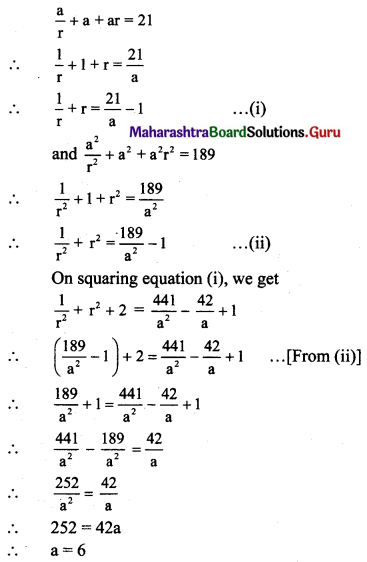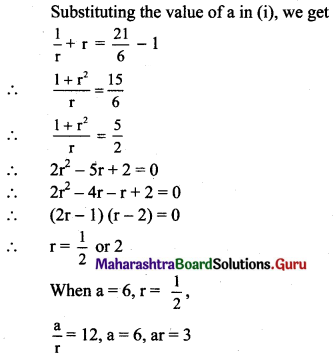When a = 6, r = 2,
$$\frac{a}{r}$$ = 3, a = 6, ar = 12
Hence, the three numbers in G.P. are 12, 6, 3 or 3, 6, 12.Check:
If sum of the three numbers is 21 and sum of their squares is 189, then our answer is correct.
Sum of the numbers = 12 + 6 + 3 = 21
Sum of the squares of the numbers = 122 + 62 + 32
= 144 + 36 + 9
= 189

Question 7.
Find four numbers in G. P. such that the sum of the middle two numbers is $$\frac{10}{3}$$ and their product is 1.
Solution:
Let the four numbers in G.P. be $$\frac{a}{r^{3}}, \frac{a}{r}, a r, a r^{3}$$
According to the given conditions,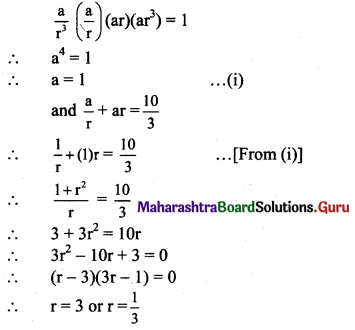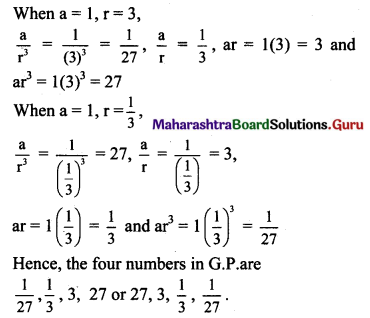Question 8.
Find five numbers in G. P. such that their product is 1024 and the fifth term is square of the third term.
Solution:
Let the five numbers in G. P. be
$$\frac{a}{r^{2}}, \frac{a}{r}, a, a r, a r^{2}$$
According to the given conditions,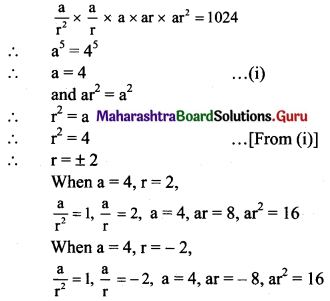Hence, the five numbers in G.P. are
1, 2, 4, 8, 16 or 1, -2, 4, -8, 16.

Question 9.
The fifth term of a G. P. is x, the eighth term of a G.P. is y and the eleventh term of a G.P. is z, verify whether y2 = xz.
Solution: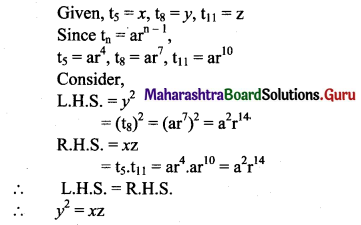Question 10.
If p, q, r, s are in G.P., show that p + q, q + r, r + s are also in G. P.
Solution:
p, q, r, s are in G.P.
∴ $$\frac{\mathrm{q}}{\mathrm{p}}=\frac{\mathrm{r}}{\mathrm{q}}=\frac{\mathrm{s}}{\mathrm{r}}$$
Let $$\frac{\mathrm{q}}{\mathrm{p}}=\frac{\mathrm{r}}{\mathrm{q}}=\frac{\mathrm{s}}{\mathrm{r}}$$ = k
∴ q = pk, r = qk, s = rk
We have to prove that p + q, q + r, r + s are in G.P.
i.e., to prove that $$\frac{\mathrm{q}+\mathrm{r}}{\mathrm{p}+\mathrm{q}}=\frac{\mathrm{r}+\mathrm{s}}{\mathrm{q}+\mathrm{r}}$$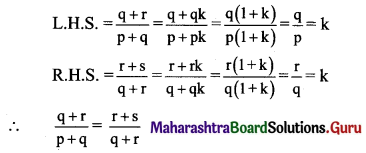∴ p + q, q + r, r + s are in G.P.Question 11.
The number of bacteria in a culture doubles every hour. If there were 50 bacteria originally in the culture, how many bacteria will be there at the end of the 5th hour?
Solution:
Since the number of bacteria in culture doubles every hour, increase in number of bacteria after every hour is in G.P.
∴ a = 50, r = $$\frac{100}{50}$$ = 2
tn = arn-1
To find the number of bacteria at the end of the 5th hour.
(i.e., to find the number of bacteria at the beginning of the 6th hour, i.e., to find t6.)
∴ t6 = ar5
= 50 × (25)
= 50 × 32
= 1600

Question 12.
A ball is dropped from a height of 80 ft. The ball is such that it rebounds $$\left(\frac{3}{4}\right)^{\text {th }}$$ of the height it has fallen. How high does the ball rebound on the 6th bounce? How high does the ball rebound on the nth bounce?
Solution:
Since the ball rebounds $$\left(\frac{3}{4}\right)^{\text {th }}$$ of the height it has fallen, the height in successive bounce is in G.P.
1st height in the bounce = 80 × $$\frac{3}{4}$$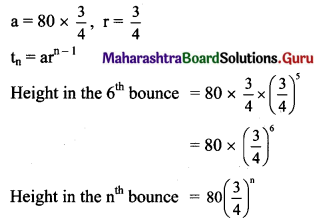Question 13.
The numbers 3, x and x + 6 are in G. P. Find
(i) x
(ii) 20th term
(iii) nth term.
Solution:
(i) 3, x and x + 6 are in G. P.
$$\frac{x}{3}=\frac{x+6}{x}$$
x2 = 3x + 18
x2 – 3x – 18 = 0
(x – 6) (x + 3) = 0
x = 6, -3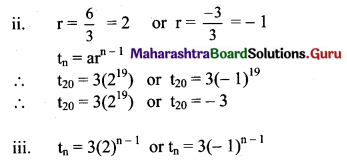Question 14.
Mosquitoes are growing at a rate of 10% a year. If there were 200 mosquitoes in the beginning, write down the number of mosquitoes after
(i) 3 years
(ii) 10 years
(iii) n years
Solution:
a = 200, r = 1 + $$\frac{10}{100}$$ = $$\frac{11}{10}$$
Mosquitoes at the end of 1st year = 200 × $$\frac{11}{10}$$
(i) Number of mosquitoes after 3 years
= 200 × $$\frac{11}{10} \times\left(\frac{11}{10}\right)^{2}$$
= 200 $$\left(\frac{11}{10}\right)^{3}$$
= 200 (1.1)3

(ii) Number of mosquitoes after 10 years = 200 (1.1)10

(iii) Number of mosquitoes after n years = 200 (1.1)n

Question 15.
The numbers x – 6, 2x and x2 are in G. P. Find
(i) x
(ii) 1st term
(iii) nth term
Solution:
(i) x – 6, 2x and x are in Geometric progression.
∴ $$\frac{2 x}{x-6}=\frac{x^{2}}{2 x}$$
4x2 = x2(x – 6)
4 = x – 6
x = 10(ii) t1 = x – 6 = 10 – 6 = 4